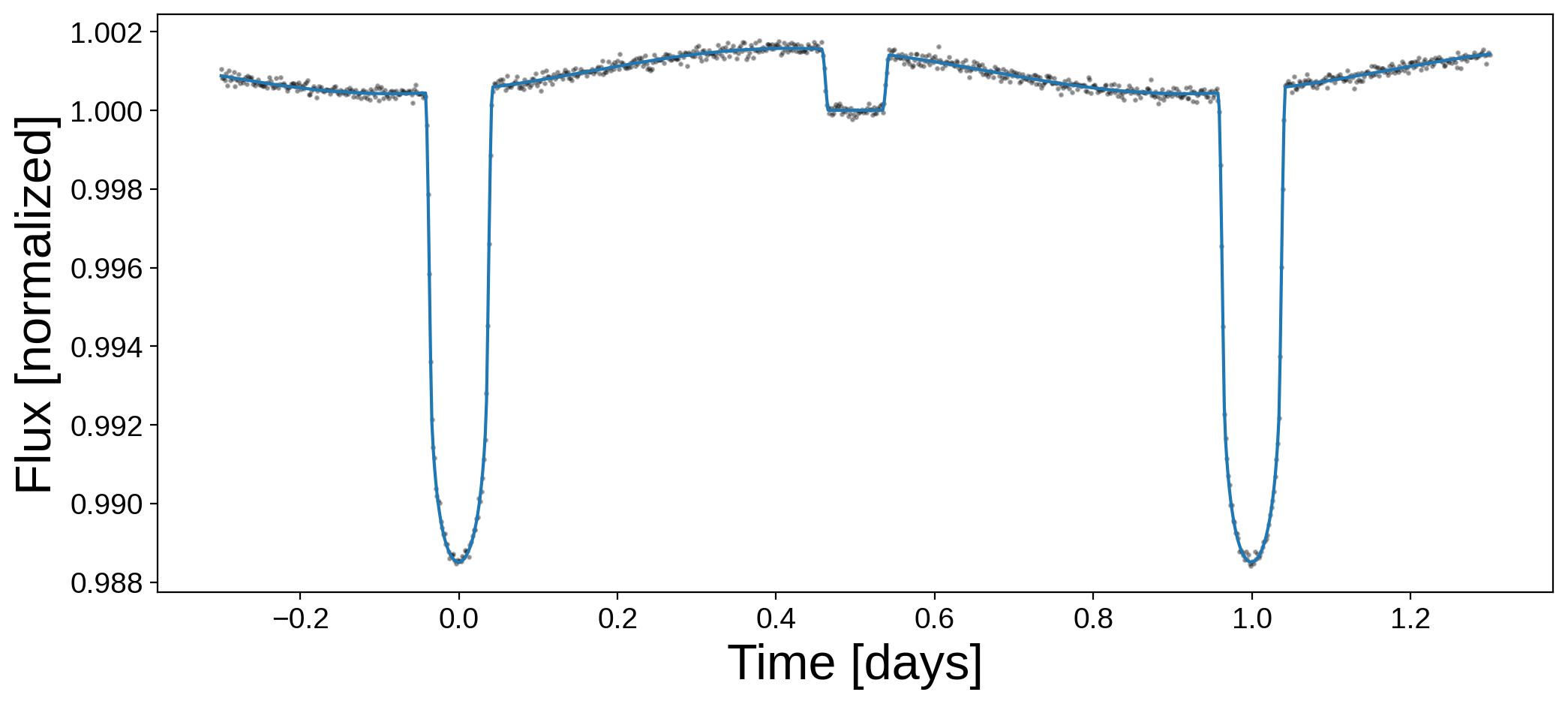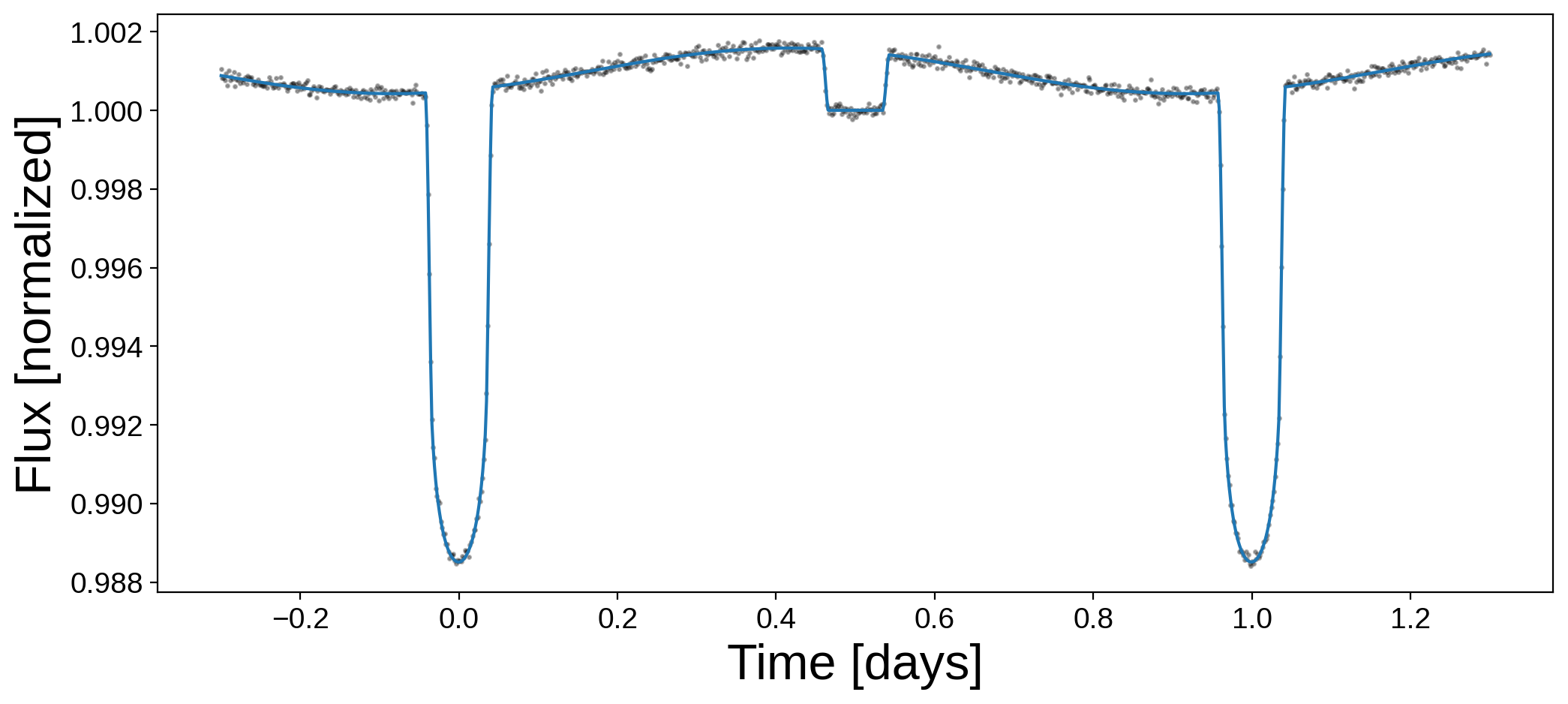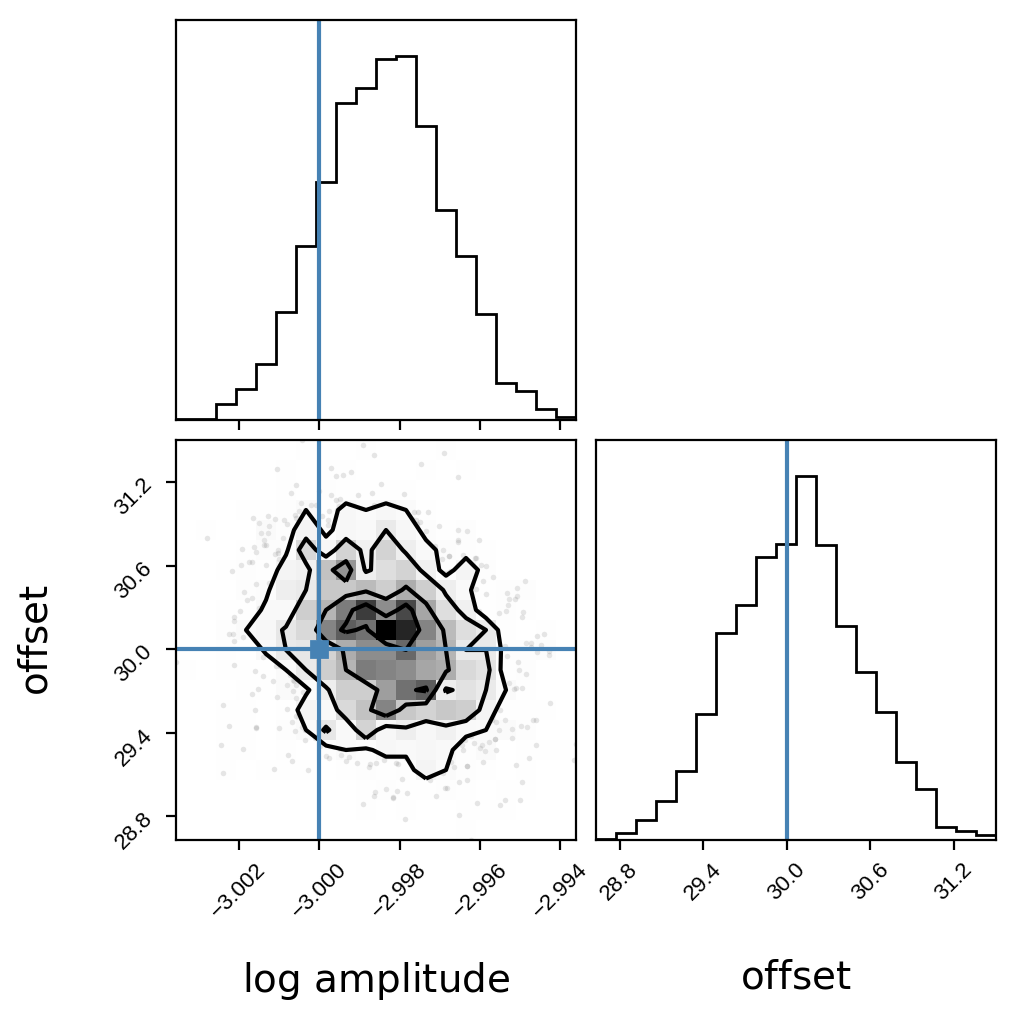Note

This tutorial was generated from a Jupyter notebook that can be downloaded here.

# Hot jupiter phase curve example¶

In this notebook, we’ll run through a brief example of how to model a full hot jupiter light curve – including the transit, secondary eclipse, and phase curve – using the machinery of the exoplanet package.

Let’s begin with our custom imports. Note that we want to run starry in lazy mode (the default), since we need to be able to compute analytic derivatives of the model for use in pymc3.

:

import starry
import matplotlib.pyplot as plt
import numpy as np
import pymc3 as pm
import pymc3_ext as pmx
import exoplanet

starry.config.quiet = True
np.random.seed(1)


## Generating a dataset¶

Let’s generate some synthetic data. First we create a star…

:

A = starry.Primary(starry.Map(ydeg=0, udeg=2, amp=1.0), m=1.0, r=1.0, prot=1.0)
A.map = 0.4
A.map = 0.2


… and now we instantiate the planet…

:

# These are the parameters we're going to try to infer
log_amp_true = -3.0
offset_true = 30.0

b = starry.Secondary(
starry.Map(ydeg=1, udeg=0, amp=10 ** log_amp_true, inc=90.0, obl=0.0),
m=0.0,
r=0.1,
inc=90.0,
prot=1.0,
porb=1.0,
)
b.map[1, 0] = 0.5
b.theta0 = 180.0 + offset_true


Most of the parameters should be self-explanatory (check the docs for details). For the planet, we give it a simple dipole map by setting only the $$Y_{1,0}$$ coefficient. We then set the theta0 parameter to be $$180^\circ$$ plus an offset, which we set to be $$30^\circ$$. The parameter theta0 is the rotational phase of the map at the reference time t0, which in this case is the time of transit. For a tidally-locked close-in planet, we usually want the bright side of the map to be facing the star at that point, which we accomplish by setting theta0=180. The offset captures the misalignment between the hot spot of the planet and the sub-stellar point, as is seen in the hot jupiter HD 189733b. In this notebook, we’ll attempt to solve for this value.

Next, we instantiate the system:

:

sys = starry.System(A, b)


We can now generate a synthetic light curve, and add some noise:

:

t = np.linspace(-0.3, 1.3, 1000)
flux_true = sys.flux(t).eval()
ferr = 1e-4
flux = flux_true + ferr * np.random.randn(len(t))
plt.figure(figsize=(12, 5))
plt.plot(t, flux, "k.", alpha=0.3, ms=3)
plt.plot(t, flux_true)
plt.xlabel("Time [days]", fontsize=24)
plt.ylabel("Flux [normalized]", fontsize=24);By eye we can tell there’s an offset, since the peak in the phase curve does not coincide with the secondary eclipse.

## Fitting the data¶

We’re going to fit this light curve using exoplanet and pymc3. Let’s begin fresh and define a new star, planet, and system, this time within a pymc3 model context:

:

with pm.Model() as model:

# These are the variables we're solving for;
# here we're placing wide Gaussian priors on them.
offset = pm.Normal("offset", 0.0, 50.0, testval=0.11)
log_amp = pm.Normal("log_amp", -4.0, 2.0, testval=-3.91)

# Instantiate the star; all its parameters are assumed
# to be known exactly
A = starry.Primary(
starry.Map(ydeg=0, udeg=2, amp=1.0, inc=90.0, obl=0.0), m=1.0, r=1.0, prot=1.0
)
A.map = 0.4
A.map = 0.2

# Instantiate the planet. Everything is fixed except for
# its luminosity and the hot spot offset.
b = starry.Secondary(
starry.Map(ydeg=1, udeg=0, amp=10 ** log_amp, inc=90.0, obl=0.0),
m=0.0,
r=0.1,
prot=1.0,
porb=1.0,
)
b.map[1, 0] = 0.5
b.theta0 = 180.0 + offset

# Instantiate the system as before
sys = starry.System(A, b)

# Our model for the flux
flux_model = pm.Deterministic("flux_model", sys.flux(t))

# This is how we tell pymc3 about our observations;
# we are assuming they are ampally distributed about
# the true model. This line effectively defines our
# likelihood function.
pm.Normal("obs", flux_model, sd=ferr, observed=flux)


Great! The first thing we usually do is run this model through an optimizer (which is usually fast, since starry computes derivatives):

:

with model:
map_soln = pmx.optimize()

optimizing logp for variables: [log_amp, offset]

100.00% [77/77 00:00<00:00 logp = 7.803e+03]


message: Desired error not necessarily achieved due to precision loss.
logp: -26221.397786421036 -> 7803.390365698361


Here’s what our best model looks like:

:

plt.figure(figsize=(12, 5))
plt.plot(t, flux, "k.", alpha=0.3, ms=3)
plt.plot(t, map_soln["flux_model"])
plt.xlabel("Time [days]", fontsize=24)
plt.ylabel("Flux [normalized]", fontsize=24);And here are the best-fit values of the two parameters:

:

print("offset:", map_soln["offset"])
print("log_amp:", map_soln["log_amp"])

offset: 30.052031477548603
log_amp: -2.998333491714684


Not bad! If we just cared about finding the best solution, we’d be done, but we actually want posteriors over the model parameters. For this, we’re going to do sampling with pymc3:

:

with model:
trace = pmx.sample(
tune=250,
draws=500,
start=map_soln,
chains=4,
cores=1,
target_accept=0.9,
)

Sequential sampling (4 chains in 1 job)
NUTS: [log_amp, offset]

100.00% [750/750 00:18<00:00 Sampling chain 0, 0 divergences]
100.00% [750/750 00:18<00:00 Sampling chain 1, 0 divergences]
100.00% [750/750 00:18<00:00 Sampling chain 2, 0 divergences]
100.00% [750/750 00:19<00:00 Sampling chain 3, 0 divergences]
Sampling 4 chains for 250 tune and 500 draw iterations (1_000 + 2_000 draws total) took 75 seconds.


And we’re done! It’s usually a good idea to look at a summary of the sampling procedure:

:

pm.summary(trace, var_names=["log_amp", "offset"])

:

mean sd hdi_3% hdi_97% mcse_mean mcse_sd ess_mean ess_sd ess_bulk ess_tail r_hat
log_amp -2.998 0.002 -3.001 -2.996 0.000 0.00 1553.0 1553.0 1536.0 1218.0 1.00
offset 30.067 0.449 29.305 30.965 0.014 0.01 1048.0 1048.0 1050.0 1022.0 1.01

The mc_errors are relatively small, the Rhat convergence criterion is close to 1, and the number of effective samples n_eff is over 1000, all of which are good. We should probably run the sampler a bit longer, but this should be good enough for demonstration purposes. Let’s plot our posterior distributions:

:

import corner

samples = pm.trace_to_dataframe(trace, varnames=["log_amp", "offset"])
corner.corner(
np.array(samples),
truths=[log_amp_true, offset_true],
labels=[r"$\log\,\mathrm{amplitude}$", r"$\mathrm{offset}$"],
);Looks great! The blue lines indicate the true values.